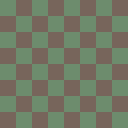## Pixel Art Tutorial: Optimizing Tilesheets

This tutorial doesn’t have that much to do with actually creating pixel art, but it’s aimed at artists smaking pixel graphics for games. It will show you how to create the best possible tilesheet layouts, without wasting any space in the image file, which is especially useful for mobile phone developers where every byte counts.

Let’s start with a small background story: when I was working for a company developing mobile games, I often had the problem of arranging tiles in a tilesheet image so that as few pixels as possible were wasted to empty space. Of course, the best “shape” for a tilesheet would simply be a very long row, only one tile high. However, this is usually not possible, because the file would become too wide for the hardware to handle. Usually, you have to deal with power of 2 image sizes, like 64, 128 or 256 pixels. This is probably mathematically the best way to solve the problem:

1. First of all you have to count the total number of tiles you have. In this example, we’ll assume we have 71 tiles, each 16×16 pixels in size.
2. Find the natural number x, which when multiplied by itself is both closest to, but also lower than your number of tiles. In our example, this number x would be 8, since 8² = 64.
3. Multiply your number x with the width and height of your tiles, in our case it would be 8*16 = 128 for both width and height.
4. Fill an image with the dimensions you just calculated with tiles. In our case, we would be able to fit 64 tiles into a 128×128 pixel tilesheet.
5.6. Expand your image’s canvas by another row of tiles, include the remaining tiles and it will leave you with a tilesheet with the least possible wasted space. In our case, it’s another 7 tiles in the last row, and only one tile that remains empty.

And that’s it. I hope the tutorial was clear enough to be easily understood and in some way useful to you.

Tags: ,

Enter this code